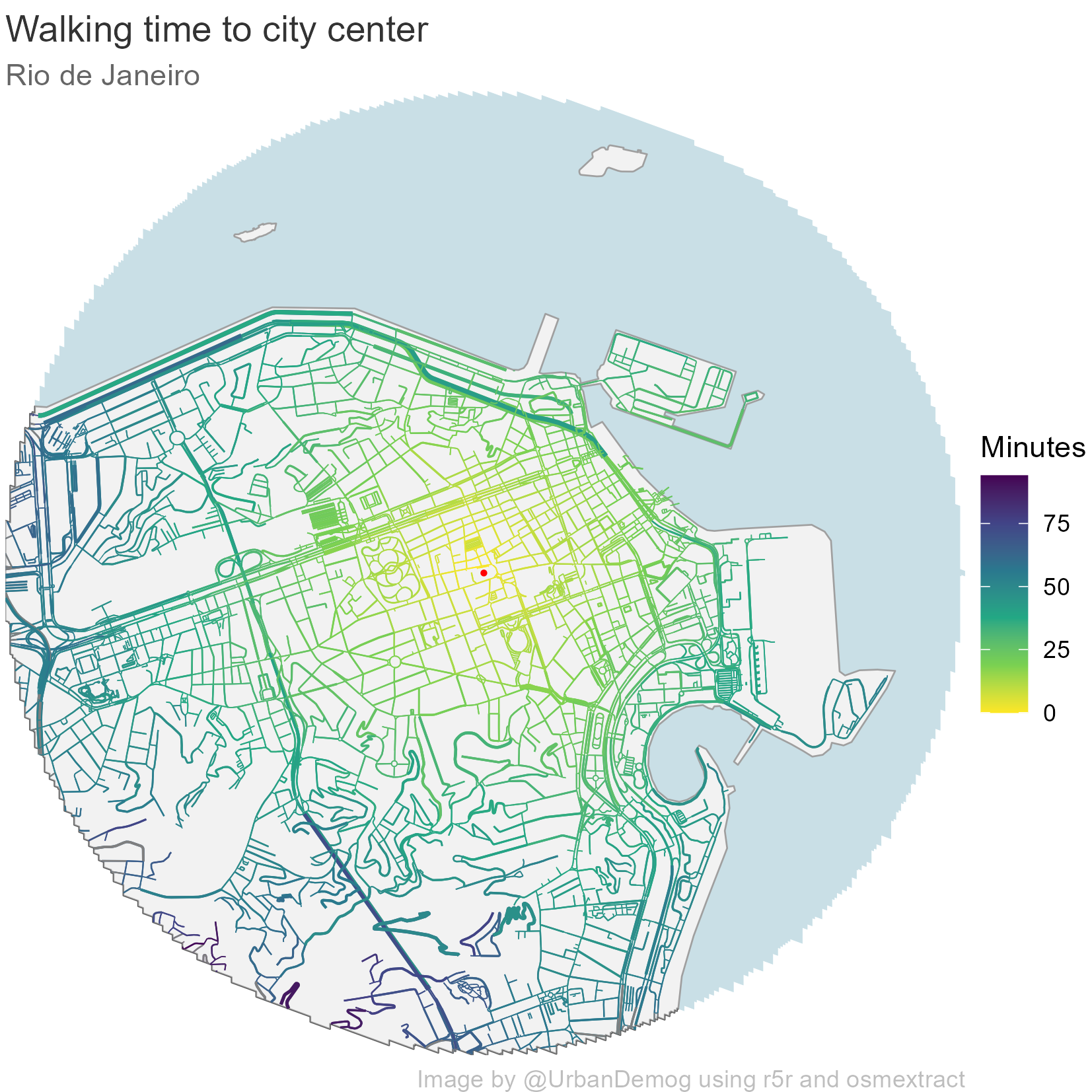## Mapping walking time on OSM data with r5r

The topic of day 5 in the #30DayMapChallenge is ‘OpenStreetMap’. So here is a quick reproducible example showing how one can use OpenStreetMap data and the r5r package to calculate travel times.

``````options(java.parameters = "-Xmx4G")

library(r5r)
library(geobr)
library(sf)
library(ggplot2)
library(here)
library(osmextract)
library(data.table)
library(magrittr)

# create subdirectories "data" and "img"
dir.create(here::here("data"))
dir.create(here::here("img"))

# get city boundaries
city <- 'Rio de Janeiro'
city_code <- lookup_muni(name_muni = city)
city_boundary <- read_municipality(code_muni = city_code\$code_muni, simplified = F)

# define city center
city_center_df <- data.frame(id='center', lon=-43.182811, lat=-22.906906)
city_center_sf <- sfheaders::sfc_point(obj = city_center_df, x='lon', y='lat')
st_crs(city_center_sf) <- 4326

# define buffer area of analysis 3 Km
buff <- st_buffer(city_center_sf, dist = 3000)

# crs
city_boundary <- st_transform(city_boundary, 4326)
city_center_sf <- st_transform(city_center_sf, 4326)
buff_bb <- st_bbox(buff)

# get OSM data
file_url = osmextract::oe_match("Rio De Janeiro")[],

# build routing network
r5r_core <- r5r::setup_r5(data_path = here::here("data"), verbose = FALSE)

# get street network as sf
street_network <- r5r::street_network_to_sf(r5r_core)

# drop network outside our buffer
edges_buff <- street_network\$edges[buff, ] %>% st_intersection(., buff)
vertices_buff <- street_network\$vertices[buff, ] %>% st_intersection(., buff)
city_boundary_buff <- st_intersection(city_boundary, buff)
plot(city_boundary_buff)

vertices_buff\$id <- vertices_buff\$index

# calculate travel times to city center
tt <- r5r::travel_time_matrix(r5r_core,
origins = vertices_buff,
destinations = city_center_df,
mode = 'walk')

# add travel time info to street network
tt\$fromId <- as.numeric(tt\$fromId)
setDT(edges_buff)[tt, on=c('from_vertex'='fromId'), travel_time := i.travel_time]
edges_buff <- st_sf(edges_buff)

``````

Now we only need to plot and save the figure.

``````# figure
temp <- ggplot() +
geom_sf(data=buff, fill='lightblue', color=NA)  +
geom_sf(data=city_boundary_buff, fill='white', size=.3)  +
geom_sf(data=buff, fill='gray90', color=NA, alpha=.5)  +
geom_sf(data=edges_buff, aes(color=travel_time, size=length))  +
geom_sf(data=city_center_sf, color='red', size=.5) +
scale_size_continuous(range = c(0.1, .8), guide='none') +
scale_color_viridis_c(direction = -1) +
labs(title='Walking time to city center',
subtitle = 'Rio de Janeiro',
caption = 'Image by @UrbanDemog using r5r and osmextract',
color='Minutes') +
coord_sf(xlim = c(buff_bb[], buff_bb[]),
ylim = c(buff_bb[], buff_bb[]), expand = FALSE) +
theme_void() +
theme(plot.background = element_rect(fill = "white", color='white'),
plot.title = element_text(color = "gray20"),
plot.subtitle = element_text(color = "gray40"),
plot.caption = element_text(color = "gray"))

# save figure
ggsave(temp, file=here::here("img", "aa32k.png"),
dpi=300, width = 14, height = 14, units = 'cm')

``````